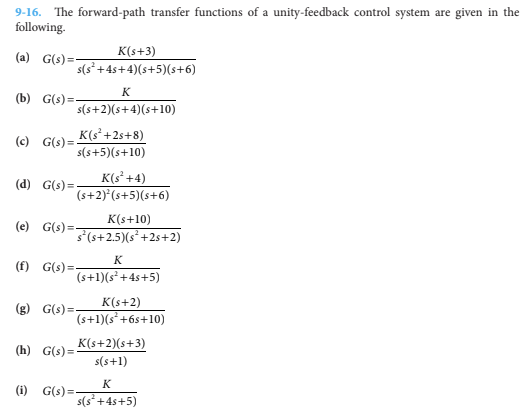# Question Construct the root loci for K ≥ 0. Find the value of K that makes the relative damping ratio of the closed-loop system (measured by the dominant complex characteristic equation roots) equal to 0.707, if such a solution exists. please solve (d) 9-16. The forward-path transfer functions of a unity-feedback control system are given in the following (a) G(s) = K(s+3) s(s* + 4s+4)(s+5)(s+6) K (b) G(s)=- s(s+2)(s+4)(s+10) K(s? +2s+8) (c) G(s)= s(s+5)(s+10) K(s?+4) (d) G(s) = (s+2)*(s+5)(+6) (e) G(s)= K(+10) s(s+2.5)(s' +2s+2) K (f) G(s) =- (s+1)(s* +45+5) K(s+2) (g) G(s)=- (s+1)(s* +6s+10) (h) G(s) = K(s+2)(s+3) s(s+1) K (i) G(s)= s(s? +4s+5)C7IDN0 The Asker · Electrical EngineeringConstruct the root loci for K ≥ 0. Find the value of K that makes the relative damping ratio of the
closed-loop system (measured by the dominant complex characteristic equation roots) equal to 0.707,
if such a solution exists.